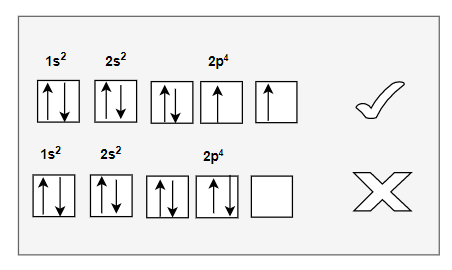# Hund's rule of maximum multiplicity

## Hund's rule of maximum multiplicity

• Pauli Exclusion rule shows that we cannot have more than 2 electrons in orbital and Also aufbau rules tells us that electron are filled up in increasing order of energy level. But both of these rules does not tell any thing how electrons would be arrange in p-orbital or d-orbital.
• Lets take an example of Carbon (C=6). If we follow Aufbau rule and Pauli exclusion rule, we will have two electrons in 2p subshell. Now arrange of those two electron can be any of these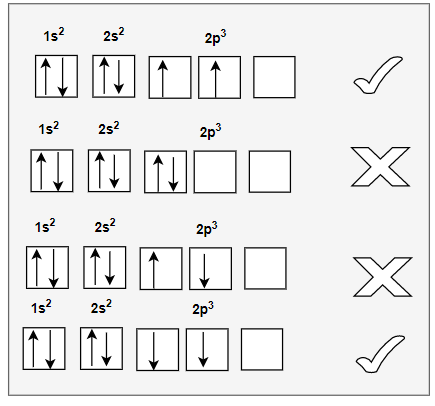Hund's rule identifies the first and last option as the electronic ground state.
• This rule deals with the filling of electrons into the orbitals belonging to the same subshell
• It states that pairing of electrons in the orbital’s belonging to same sub – shell [ p, d, f], does not take place until each orbital gets singly occupied.
• All the orbitals which are singly occupied have parallel spins which can be either clockwise or anticlockwise.Electrons have parallel spin have less inter-electronic repulsions and high spin multiplicity. The reason is that Electrons are negatively charged and, as a result, they repel each other. Electrons tend to minimize repulsion by occupying their own orbitals, rather than sharing an orbital with another electron.
• Now we have three orbitals in p subshell,so pairing or doubling of electrons will start from 4th entry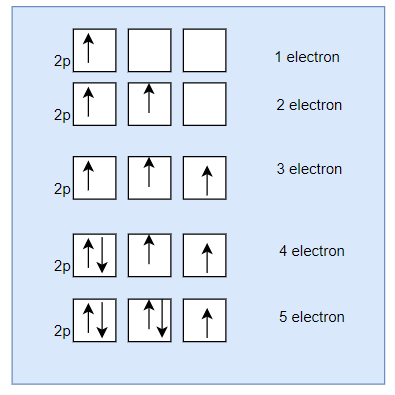• Similarly we have five d orbitals, therefore, the pairing of electrons will start in the 6th electron only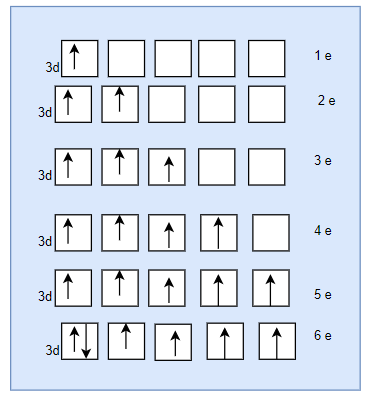• Now we have 7 orbitals in f subshell,so doubling of electrons will start from 8th electron only

## Examples

a. Nitrogen (N) , Z=7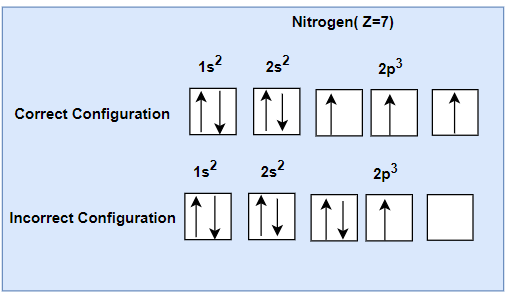We can observe that there are three electrons in p subshell.The p orbitals are half-filled with one electron in each of the orbital.
b. Nitrogen (O) , Z=8